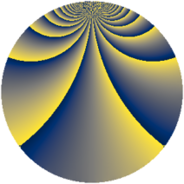# Properties

 Label 507.2.kLevel $507$ Weight $2$ Character orbit 507.k Rep. character $\chi_{507}(80,\cdot)$ Character field $\Q(\zeta_{12})$ Dimension $164$ Newform subspaces $11$ Sturm bound $121$ Trace bound $7$

# Related objects

## Defining parameters

 Level: $$N$$ $$=$$ $$507 = 3 \cdot 13^{2}$$ Weight: $$k$$ $$=$$ $$2$$ Character orbit: $$[\chi]$$ $$=$$ 507.k (of order $$12$$ and degree $$4$$) Character conductor: $$\operatorname{cond}(\chi)$$ $$=$$ $$39$$ Character field: $$\Q(\zeta_{12})$$ Newform subspaces: $$11$$ Sturm bound: $$121$$ Trace bound: $$7$$ Distinguishing $$T_p$$: $$2$$, $$5$$, $$7$$

## Dimensions

The following table gives the dimensions of various subspaces of $$M_{2}(507, [\chi])$$.

Total New Old
Modular forms 300 244 56
Cusp forms 188 164 24
Eisenstein series 112 80 32

## Trace form

 $$164 q + 2 q^{3} + 12 q^{4} + 2 q^{6} + 14 q^{7} + 2 q^{9} + O(q^{10})$$ $$164 q + 2 q^{3} + 12 q^{4} + 2 q^{6} + 14 q^{7} + 2 q^{9} - 12 q^{10} + 14 q^{15} + 4 q^{16} - 4 q^{18} + 2 q^{19} - 22 q^{21} - 12 q^{22} - 18 q^{24} - 76 q^{27} - 18 q^{30} + 6 q^{31} - 16 q^{33} + 36 q^{34} + 36 q^{36} + 30 q^{37} - 56 q^{40} + 24 q^{42} - 30 q^{43} + 20 q^{45} + 106 q^{48} - 18 q^{49} - 46 q^{54} + 4 q^{55} - 28 q^{57} - 28 q^{58} - 44 q^{60} - 36 q^{61} - 16 q^{63} + 8 q^{67} + 32 q^{70} - 12 q^{72} + 62 q^{73} + 18 q^{75} + 36 q^{76} - 112 q^{79} + 14 q^{81} + 24 q^{82} + 8 q^{84} - 12 q^{85} - 22 q^{87} + 12 q^{88} - 10 q^{93} - 112 q^{94} - 16 q^{96} + 18 q^{97} - 40 q^{99} + O(q^{100})$$

## Decomposition of $$S_{2}^{\mathrm{new}}(507, [\chi])$$ into newform subspaces

Label Dim $A$ Field CM Traces $q$-expansion
$a_{2}$ $a_{3}$ $a_{5}$ $a_{7}$
507.2.k.a $4$ $4.048$ $$\Q(\zeta_{12})$$ $$\Q(\sqrt{-3})$$ $$0$$ $$0$$ $$0$$ $$-8$$ $$q+(\zeta_{12}+\zeta_{12}^{3})q^{3}+2\zeta_{12}q^{4}+(-1+\cdots)q^{7}+\cdots$$
507.2.k.b $4$ $4.048$ $$\Q(\zeta_{12})$$ $$\Q(\sqrt{-3})$$ $$0$$ $$0$$ $$0$$ $$8$$ $$q+(\zeta_{12}+\zeta_{12}^{3})q^{3}+2\zeta_{12}q^{4}+(1+\zeta_{12}+\cdots)q^{7}+\cdots$$
507.2.k.c $4$ $4.048$ $$\Q(\zeta_{12})$$ $$\Q(\sqrt{-3})$$ $$0$$ $$0$$ $$0$$ $$10$$ $$q+(-\zeta_{12}-\zeta_{12}^{3})q^{3}+2\zeta_{12}q^{4}+(2+\cdots)q^{7}+\cdots$$
507.2.k.d $8$ $4.048$ 8.0.56070144.2 None $$0$$ $$-2$$ $$0$$ $$4$$ $$q+(-\beta _{1}-\beta _{2}+\beta _{3}-\beta _{4}+2\beta _{5}+\beta _{7})q^{2}+\cdots$$
507.2.k.e $8$ $4.048$ 8.0.56070144.2 None $$0$$ $$-2$$ $$0$$ $$-4$$ $$q+(\beta _{2}-\beta _{5}-\beta _{7})q^{2}+(-\beta _{2}+\beta _{4})q^{3}+\cdots$$
507.2.k.f $8$ $4.048$ 8.0.56070144.2 None $$0$$ $$-2$$ $$0$$ $$4$$ $$q+(\beta _{2}-\beta _{5}-\beta _{7})q^{2}+(\beta _{2}+\beta _{4}-\beta _{5}+\cdots)q^{3}+\cdots$$
507.2.k.g $8$ $4.048$ 8.0.56070144.2 $$\Q(\sqrt{-39})$$ $$0$$ $$0$$ $$0$$ $$0$$ $$q-\beta _{6}q^{2}+(-\beta _{2}-2\beta _{3})q^{3}+(-2+2\beta _{3}+\cdots)q^{4}+\cdots$$
507.2.k.h $8$ $4.048$ 8.0.56070144.2 $$\Q(\sqrt{-39})$$ $$0$$ $$0$$ $$0$$ $$0$$ $$q+\beta _{5}q^{2}+(2\beta _{2}+\beta _{3})q^{3}+(1+2\beta _{2}+\cdots)q^{4}+\cdots$$
507.2.k.i $8$ $4.048$ $$\Q(\zeta_{24})$$ None $$0$$ $$4$$ $$0$$ $$4$$ $$q+\zeta_{24}^{7}q^{2}+(\zeta_{24}+\zeta_{24}^{4}-\zeta_{24}^{7})q^{3}+\cdots$$
507.2.k.j $8$ $4.048$ $$\Q(\zeta_{24})$$ None $$0$$ $$4$$ $$0$$ $$-4$$ $$q+\zeta_{24}^{7}q^{2}+(-\zeta_{24}+\zeta_{24}^{4}+\zeta_{24}^{7})q^{3}+\cdots$$
507.2.k.k $96$ $4.048$ None $$0$$ $$0$$ $$0$$ $$0$$

## Decomposition of $$S_{2}^{\mathrm{old}}(507, [\chi])$$ into lower level spaces

$$S_{2}^{\mathrm{old}}(507, [\chi]) \cong$$ $$S_{2}^{\mathrm{new}}(39, [\chi])$$$$^{\oplus 2}$$